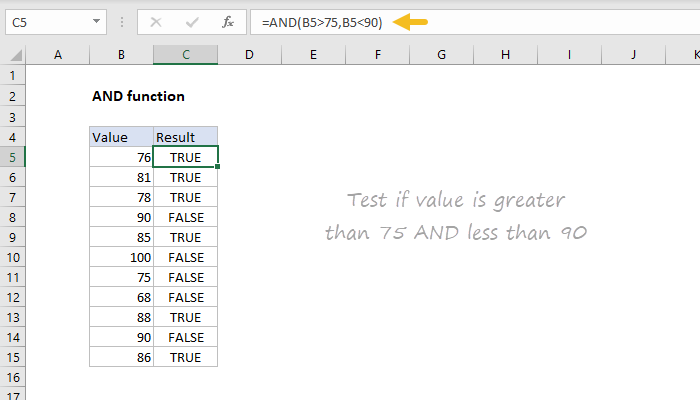Purpose
Test multiple conditions with AND

Return value
TRUE if all arguments evaluate TRUE; FALSE if not

Arguments
logical1 - The first condition or logical value to evaluate.
logical2 - [optional] The second condition or logical value to evaluate.

Syntax
=AND(logical1, [logical2], ...)

To test if the value in A1 is greater than 0 and less than 5, you can use AND like this:
=AND(A1>0,A1<5)

You can embed the AND function inside the IF function. Using the above example, you can supply AND as the logical_test for the IF function like so:
=IF(AND(A1>0,A1<5), "Approved", "Denied")

This formula will return "Approved" only if the value in A1 is greater than 0 and less than 5.

You can combine the AND function with the OR function. The formula below returns TRUE when A1 > 100 and B1 is "complete" or "pending":
=AND(A1>100,OR(B1="complete",B1="pending"))